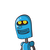# The length and breadth of a rectangle are in the ratio 3:2 Ifthe breadth is 2a , and the perimeter of the rectangle is 50 October 18, 2021 by Lyla

The length and breadth of a rectangle are in the ratio 3:2 If
the breadth is 2a , and the perimeter of the rectangle is 50
centimeter find its length and breadth​

### 1 thought on “The length and breadth of a rectangle are in the ratio 3:2 If<br />the breadth is 2a , and the perimeter of the rectangle is 50<br”

1.Step-by-step explanation:

Let the length of rectangle = 3x